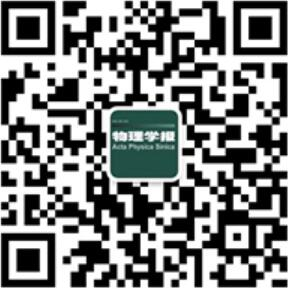#### 搜索

x## 留言板## 基于紧束缚模型的拓扑物理微波实验验证平台的开发

PDF
HTML
• #### 摘要

拓扑光子学、拓扑物理与光学的结合, 为凝聚态理论的验证以及新型光学器件的构建提供了新的视角. 紧束缚模型是凝聚态物理的重要研究手段. 我们发现, 将传统光子晶体的背景材料由通常的空气改为有效介电常数为负数的材料之后, 这样的光子晶体和紧束缚模型有一一对应的关系, 可以用于相关理论的验证. 通过数值仿真实验, 在蜂巢晶格负背景光子晶体结构中验证了之字形(zigzag)、胡须型(bearded)等界面态的存在性. 我们提出了两种实验构想, 以期在微波频段开发相应的凝聚态理论验证平台, 为拓扑物理的研究提供全新的工具. 我们也希望, 这些新理论的验证能为今后光学仪器的设计提供崭新思路.

#### 参考文献

  Haldane F D M, Raghu S 2008 Phys. Rev. Lett. 100 013904Poo Y, Wu R X, Lin Z, Yang Y, Chan C T 2011 Phys. Rev. Lett. 106 093903Wang Z, Chong Y, Joannopoulos J D, Soljačić M 2009 Nature 461 772Ashcroft N W, Mermin N D, Solid State Physics, 1976, Thomson Learning Asia Pte Ltd.  Bernevig B A, Hughes T L, Topological insulators and topological superconductors, 2013, Princeton University Press, Princeton, NJ.  Lu L, Joannopoulos J D, Soljačić M 2016 Nat. Phys. 12 626Xiao S, Zhong F, Liu H, Zhu S, Li J 2015 Nat. Commun. 6 8360胡中, 徐涛, 汤蓉, 郭会杰 2019 激光与光电子学进展 56 202408 Hu Z, Xu T, Tang R, Guo H J 2019 Laser & Optoelectronics Progress 56 202408  Sun S, He Q, Xiao S, Xu Q, Li X, Zhou L 2012 Nat. Mater. 11 426Joannopoulos J D, Johnson S G, Winn J N, Meade R D, Photonic crystals: molding the flow of light, 2 nd ed. 2008, Princeton University Press, Princeton, NJ.  Lidorikis E, Sigalas M M, Economou E N, Soukoulis C M 1998 Phys. Rev. Lett. 81 1405Plotnik Y, Rechtsman M C, Song D, Heinrich M, Zeuner J M, Nolte S, Lumer Y, Malkova N, Xu J, Szameit A, Chen Z, Segev M 2014 Nat. Mater. 13 57Pendry J B, Holden A J, Stewart W J, Youngs I 1996 Phys. Rev. Lett. 76 4773Simovski C R, Belov P A, Atrashchenko A V, Kivshar Y S 2012 Adv. Mater. 24 4229Belov P A, Marques R, Maslovski S I, Nefedov I S, Silveirinha M, Simovski C R, Tretyakov S A 2003 Phys. Rev. B 67 113103Wang B, Cappelli M A 2016 Appl. Phys. Lett. 108 161101Wang B, Cappelli M A 2015 Appl. Phys. Lett. 107 171107Skirlo S, Lu L, Igarashi L, Yan Q, Joannopoulos J D, Soljačić M 2015 Phys. Rev. Lett. 115 253901Khanikaev A B 2013 Nat. Mater. 12 233Ye L, Yang Y, Hang Z H, Qiu C, Liu Z 2017 Appl. Phys. Lett. 111 251107Yang Y, Xu Y F, Xu T, Wang H, Jiang J, Hu X, Hang Z H 2018 Phys. Rev. Lett. 120 217401He X, Liang E, Yuan J, Qiu H, Chen X, Zhao F, Dong J 2019 Nat. Commun. 10 872#### 施引文献

• 图 1  二维类石墨烯光子晶体结构示意图, 其蜂巢晶格原胞用红线标记. y轴方向上11个介质柱构成一个超原胞, 沿着x轴无限周期排列. 介质柱阵列的S1边界为之字形(zigzag)界面, S2为胡须形(bearded)界面, 介质柱半径r = 0.2a, a是蜂巢晶格的晶格常数

Fig. 1.  The structure of a honeycomb-lattice graphene-like photonic crystal. Its honeycomb unit cell is indicated in red. A ribbon of 11 dielectric cylinders along the y direction is considered and it is periodically arranged in the x direction. The photonic crystal ribbon has zigzag (S1), bearded (S2) edges. The radius of cylinders shown here is r = 0.2a, where a is the lattice constant.

图 2  (a)六角光子晶体最低的两条能带, 在布里渊区边界K点因为蜂巢晶格对称性具有狄拉克点. Band 0 对应了x = –10, y = 0.2, z = 8.5的能带, 即初始条件. Band x, y, z 在保持另两个初始值不变时, 改变了x = –5 (band x), y = 0.18 (band y), z = 10 (band z); (b) 左侧band (x = –20, y = 0.2, z = 8.5)和右侧band (x = –15, y = 0.18, z = 8.5) 对比了COMSOL计算的能带(点)以及用(5)式和(6)式拟合的紧束缚模型计算得到的能带(三角形)

Fig. 2.  (a) A Dirac cone dispersion appears at K point for the lowest two photonic bands of the honeycomb photonic crystal structure we studied. Band 0 represents the band diagram when x = –10, y = 0.2, z = 8.5; Band x, y and z correspond to the situations that only one parameter from that of band 0 is changed: x = –5 (band x), y = 0.18 (band y), z = 10 (band z); (b) the comparison between calculated lowest two photonic bulk bands of band left (x = –20, y = 0.2, z = 8.5) and band right (x = –15, y = 0.18, z = 8.5) (in red dots) and corresponding bands calculated with tight-binding model with fitted parameters using equations (5) and (6) (in hollow blue triangles).

图 3  宽度为11且有一个之字形边界和一个胡须形边界的光子晶体类石墨烯带超原胞, 利用(1)式, (5)式和(6)式的紧束缚模型得到的能带图像(红色)和仿真结果(蓝色)的对比; 其中背景常数x = –20, 占空比y = 0.2, 超原胞如图右下角所示

Fig. 3.  The energy bands for a honeycomb-graphene-like photonic crystal ribbon (the background constant x = –20, filling ratio y = 0.2). Here we used the equations (1), (5) and (6) to derive the TB solution(red), as simulation results are marked blue. The supercell is depicted in the right-lower corner.

图 4  利用负背景光子晶体系统数值计算得到的局域在胡须界面上的两个Tamm态, 界面态的色散用红色和体带(蓝色)区分. 此时负背景介电常数为–10, 上体带和下体带各有一Tamm态; 其对应的本征场在下方显示　(a) 下体带; (b) 上体带

Fig. 4.  Band dispersion (in red dots) of Tamm-like bearded edge states calculated using photonic crystal system with background permittivity ${\epsilon}$= –10. The bulk bands are in blue dots. Their corresponding eigenfield distributions localized on bearded edge are shown in the lower panels: (a) Lower bands; (b) upper bands.

图 5  金属柱背景下, 氧化铝蜂窝结构的光子能带(红色), 和其对应的背景替换为有效介质(蓝色)后得到的能带结果符合, 但用紧束缚模型(绿色)计算得到的能带略有差别. 原胞内的大圆代表介质柱, 小圆代表密集插入的细金属柱

Fig. 5.  In the metallic background, photonic energy bands match well with the bands (Red) calculated with effective background constant (Blue). However, Tight Binding model’s result (Green) shows small variance compared to the real bands. In the schematic cell structure, bigger circle represents dielectric cylinder, while smaller circle represents thin metallic rod inserted.

表 1  参数和位能以及耦合系数的关系

Table 1.  Relationship between onsite energy, coupling ratio and parameters.

 Onsite energy ${\epsilon_0}$ t 背景材料介电常数|x| ↑ ↓ 晶体柱占空比y ↓ ↑ 晶体柱材料介电常数z ↓ ↑ ↑代表正相关, ↓代表负相关; x因为是负值, 所以这里取绝对值讨论.
•  引用本文:Citation:## 基于紧束缚模型的拓扑物理微波实验验证平台的开发

• 1. 苏州大学物理科学与技术学院, 苏州　215006
• 2. 南京大学现代工程与应用科学学院, 南京　210093

### English Abstract关注分享下载:  全尺寸图片 幻灯片